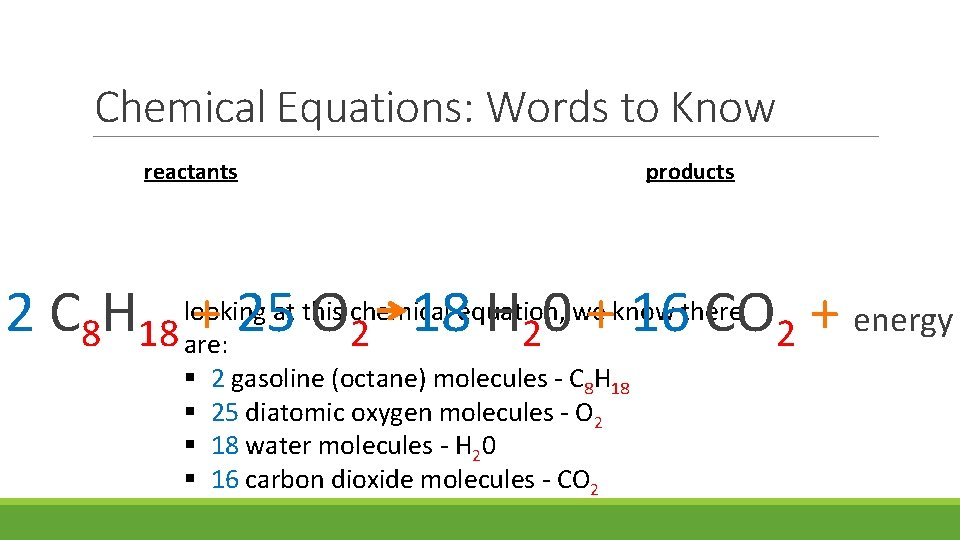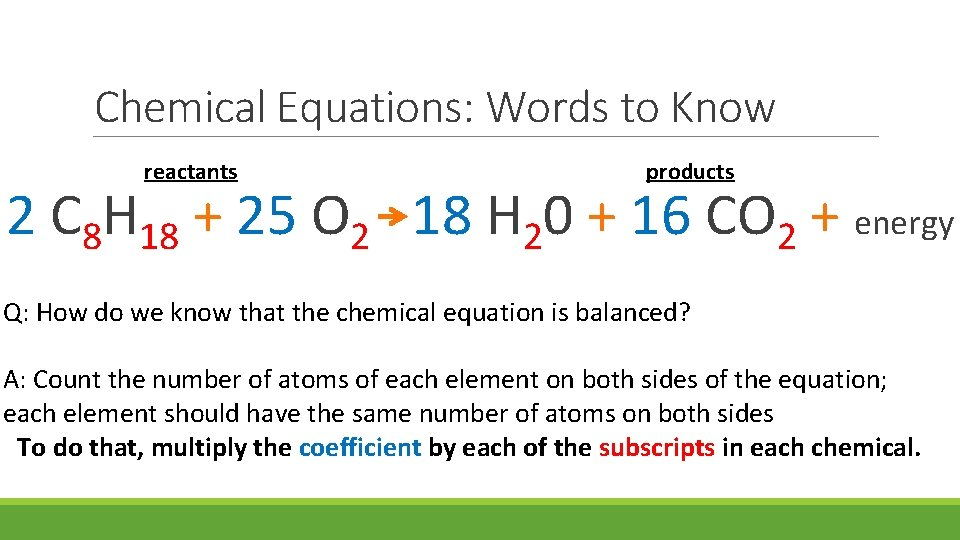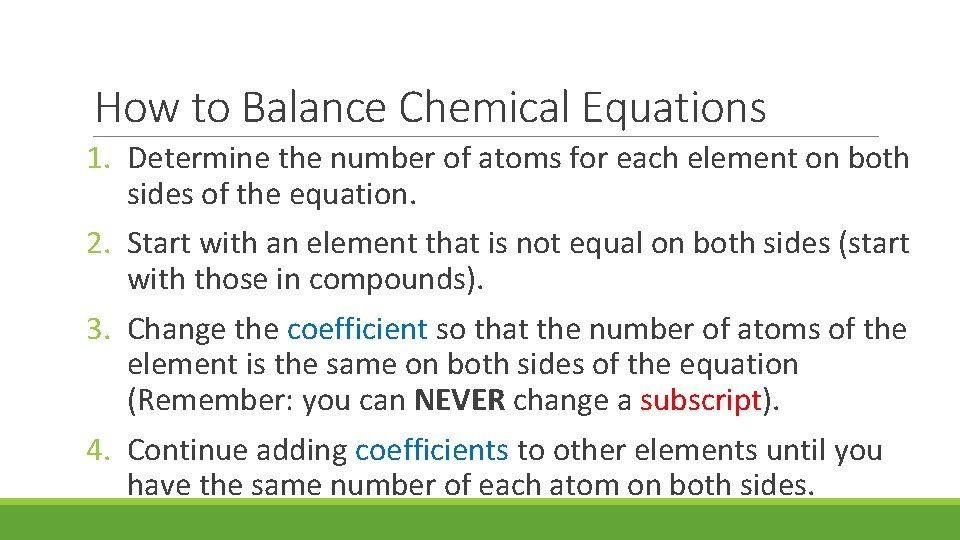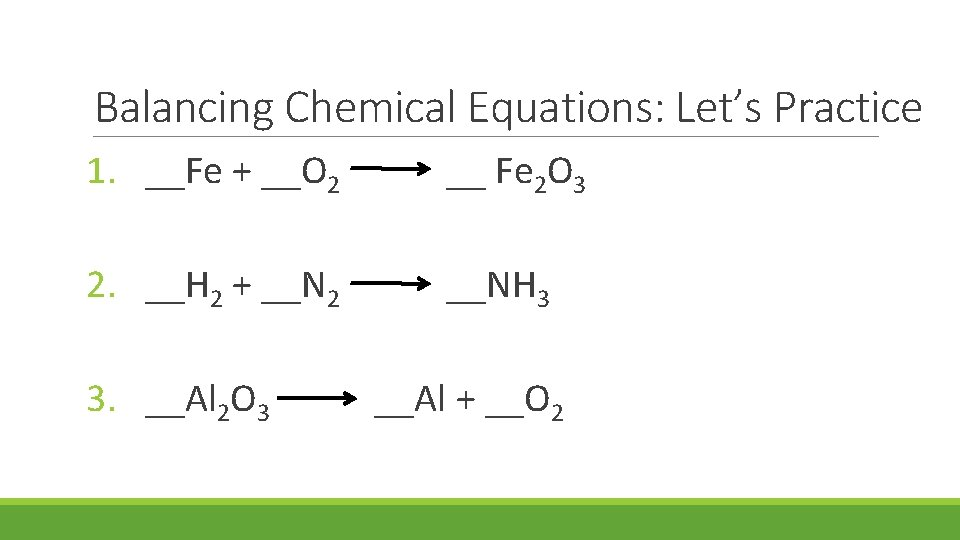# Balancing Chemical Equations Chemical Equations Review Chemical equations

• Slides: 10Balancing Chemical EquationsChemical Equations Review § Chemical equations need to be balanced due to the Law of Conservation of Mass. § This law states that matter can be changed from one form into another, but the total amount of matter stays the same. § Balancing chemical equations focuses on the number of atoms of each element involved in the chemical reaction. § Each element must have the same number of atoms on both sides of the chemical equation.Chemical Equations: Words to Know sodium bicarbonate (baking soda) Na. HCO 3 chemical name chemical formula - lists all of the elements that make up the chemical compound and the ratio of each element subscript- small number to the lower right of a symbol; identifies the number of atoms of that element in the compoundChemical Equations: Words to Know sodium bicarbonate (baking soda) Na. HCO 3 looking at the chemical formula, we know that baking soda is made of: § 1 sodium (Na) atom § 1 hydrogen (H) atom § 1 carbon (C) atom § 3 oxygen (O) atoms – O has a subscript of 3Chemical Equations: Words to Know reactants – substances you start with in a chemical rxn products – substances you end up with in a chemical rxn 2 C 8 H 18 + 25 O 2 18 H 20 + 16 CO 2 + energy gasoline (octane) reacts with oxygen to yield water + carbon dioxide + coefficient – large number in front of a chemical formula; identifies the number of molecules of each chemical energyChemical Equations: Words to Know reactants products at this chemical equation, we know there 2 C 8 H 18 looking + 25 O 18 H 0 + 16 CO + energy 2 2 2 are: § § 2 gasoline (octane) molecules - C 8 H 18 25 diatomic oxygen molecules - O 2 18 water molecules - H 20 16 carbon dioxide molecules - CO 2Chemical Equations: Words to Know reactants products 2 C 8 H 18 + 25 O 2 18 H 20 + 16 CO 2 + energy Q: How do we know that the chemical equation is balanced? A: Count the number of atoms of each element on both sides of the equation; each element should have the same number of atoms on both sides To do that, multiply the coefficient by each of the subscripts in each chemical.Chemical Equations: Words to Know reactants products 2 C 8 H 18 + 25 O 2 18 H 20 + 16 CO 2 + energy Reactants: § 16 carbon (C) atoms – 2(8) = 16 § 36 hydrogen (H) atoms – 2(18) = 36 § 50 oxygen (O) atoms – 25(2) = 50 Products: § 16 carbon (C) atoms – 16(1) = 16 § 36 hydrogen (H) atoms – 18(2) = 36 § 50 oxygen (O) atoms – 18(1) + 16(2) 18 + 32 = 50How to Balance Chemical Equations 1. Determine the number of atoms for each element on both sides of the equation. 2. Start with an element that is not equal on both sides (start with those in compounds). 3. Change the coefficient so that the number of atoms of the element is the same on both sides of the equation (Remember: you can NEVER change a subscript). 4. Continue adding coefficients to other elements until you have the same number of each atom on both sides.Balancing Chemical Equations: Let’s Practice 1. __Fe + __O 2 __ Fe 2 O 3 2. __H 2 + __N 2 __NH 3 3. __Al 2 O 3 __Al + __O 2